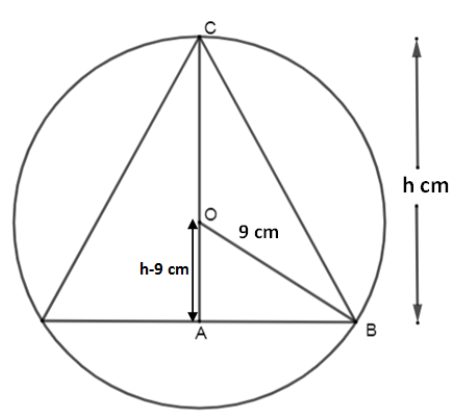The radius of a sphere is  9cm  . Find the height of the right circular cone that can be inscribed in the sphere so that the volume of the cone is maximum.

# The radius of a sphere is   . Find the height of the right circular cone that can be inscribed in the sphere so that the volume of the cone is maximum.

1. A
${h}{=}{12}{\mathit{cm}}$
2. B
3. C
${h}{=}{10}{\mathit{cm}}$
4. D
${h}{=}{11}{\mathit{cm}}$

Fill Out the Form for Expert Academic Guidance!l

+91

Live ClassesBooksTest SeriesSelf Learning

Verify OTP Code (required)

### Solution:

Let us first draw the diagram.Let the height of the cone be    and the radius of the cone be  . From the diagram, we can see that    forms a right-angled triangle. Since    represents the radius of the sphere, therefore  .
Also,    represents the radius of the sphere, therefore   .
As we can see that    and   , therefore

In right angles triangle  .
Therefore, using Pythagoras’ Theorem,

As we can see ABAB represents the radius of the base of the cone, therefore   .
Now the volume of the cone is given by ,

where    is the radius of the base of the cone and    is the height of the cone.
Therefore,
Let us take the derivative of    with respect to   . We will get,

Taking   will give us two values of   one of them gives the maximum volume of the cone and the other one gives the minimum volume of the cone.
Therefore,
gives   and  that is  .
Taking derivative of   , we get

When we put   in   , we get

,which is negative.
Therefore,    represents the maximum volume of cones inscribed in the sphere.

## Related content

 Area of Square Area of Isosceles Triangle Pythagoras Theorem Triangle Formula Perimeter of Triangle Formula Area Formulae Volume of Cone Formula Matrices and Determinants_mathematics Critical Points Solved Examples Type of relations_mathematics+91

Live ClassesBooksTest SeriesSelf Learning

Verify OTP Code (required)